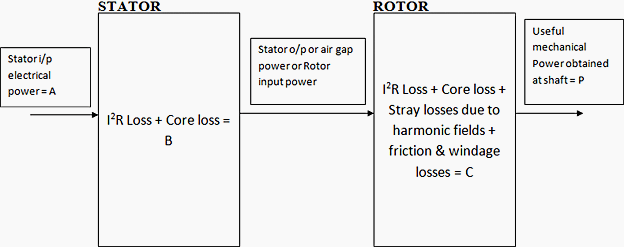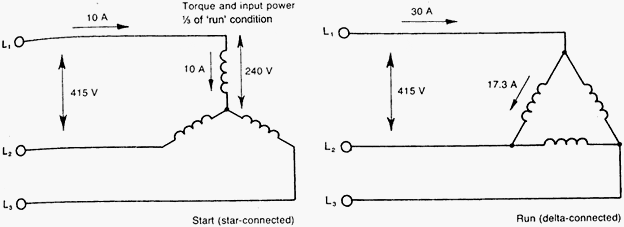## Introduction

This article will deal with those concepts of 3 phase induction motor which are essential prerequisite for proper selection, procurement, installation and maintenance of the same. Before any actual discussion on motor is started It will better to have a comparison of starting behavior of induction motor and transformer because as per the equivalent circuit representation a 3 phase induction motor is generalized transformer.
It is assumed that readers are already familiar with the elementary concept of principle of operation and construction of three phase induction motor.
What is the fundamental difference in working principle of induction motor and transformer? That is even though the equivalent circuit of motor and transformer is same rotor of motor rotates where as secondary of transformer do not.
Induction motor is a generalized transformer. Difference is that transformer is an alternating flux machine while induction motor is rotating flux machine. Rotating flux is only possible when 3 phase voltage (or poly phase) which is 120 degree apart in time is applied to a three phase winding (or poly phase winding) 120 degree apart in space then a three phase rotating magnetic flux is produced whose magnitude is constant but direction keeps changing. In transformer the flux produced is time alternating and not rotating.
There is no air gap between primary and secondary of transformer where as there is a distinct air gap between stator and rotor of motor which gives mechanical movability to motor. Because of higher reluctance ( or low permeability) of air gap the magnetizing current required in motor is 25-40% of rated current of motor where as in transformer it is only 2 -5 % of rated primary current.
In an alternating flux machine frequency of induced EMF in primary and secondary side is same where as frequency of rotor EMF depends on slip. During starting when S = 1 the frequency of induced EMF in rotor and stator is same but after loading it is not.
Other difference is that the secondary winding and core is mounted on a shaft set in bearings free to rotate and hence the name rotor.
If at all secondary of a transformer is mounted on shaft set at bearings the rate of cutting of mutual magnetic flux with secondary circuit would be different from primary and their frequency would be different. The induced EMF would not be in proportion to number of turns ratio but product of turn ratio and frequency. The ratio of primary frequency to the secondary frequency is called slip.
Any current carrying conductor if placed in magnetic field experience a force so rotor conductor experience a torque and as per Lenz’s Law the direction of motion is such that it tries to oppose the change which has caused so it starts chasing the field.

## Power flow diagram of induction motor

Stator input electrical power = A
Stator losses = B
Rotor losses = C
Mechanical output = P
A – ( B + C ) = P
Roughly B= 0.03A, C= 0.04A
A – 0.07A = P
0.93A = P, Hence efficiency = (P/A) x 100 = 93%Power flow diagram of induction motor

### Why LT motors are delta connected and HT motors are star connected?

Reason is techno commercial.
1. In star, phase current is same as line current. But phase voltage is 1/1.732 times line voltage. So insulation required in case of HT motor is less.
2. The starting current for motors is 6 to 7 times full load current. So start-up power will be large if HT motors are delta connected. It may cause instability (voltage dip) in case small Power system. In starred HT motor starting current will be less compared to delta connected motor. So starting power is reduced. Starting torque will also be reduced. (It will not be a problem as motors are of high capacity.)
3. Also as current is less copper (Cu) required for winding will be less.
4. LT motors are delta connected.
1. Insulation will not be problem as voltage level is less.
2. Starting current will not be problem as starting power in all will be less. So no problem of voltage dips.
3. Starting torque should be large, as motors are of small capacity.Comparison of star and delta motor starting
LT motors have winding delta connected.
1. In case it is having star delta starter than they are started as Star connected motor.
2. After it attains 80% of synch speed the changeover takes place from star to original configuration delta.
3. In star the voltages across the windings are lesser that is 1/1.732 times that available in delta so current is limited.
4. When it goes to delta again voltage is full line voltage so current increase even though it is lesser than the line current it remains higher than the line current drawn in star connection at reduced voltage. So cables for motor are sized for this current that is what it draws in delta connection.

#### 1 comment:

1.Bonne idée d'article, j'ai fais un article similaire ou je traîte du triphasé et des différents couplages étoile et triangle :)